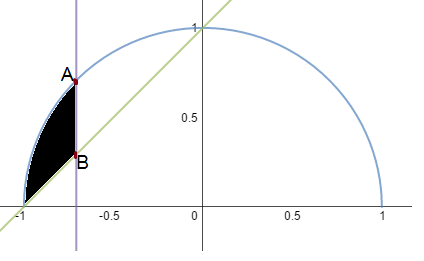# Is it a segment?The figure at right shows a unit semicircle (in blue) and the graph of $f(x)=x+1$ (in green).

Let the purple line segment $AB$ be the longest vertical line segment that joins the blue semicircle and green line in the domain $[-1,0].$

If $R$ is the area of the black shaded region, what is $\left\lfloor 100R \right\rfloor ?$

×